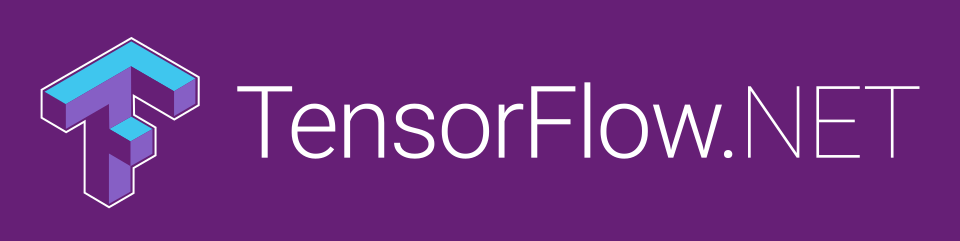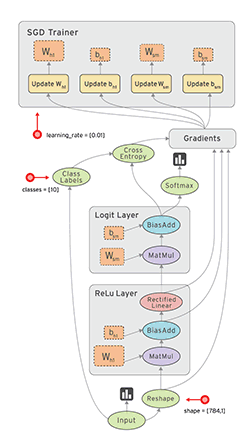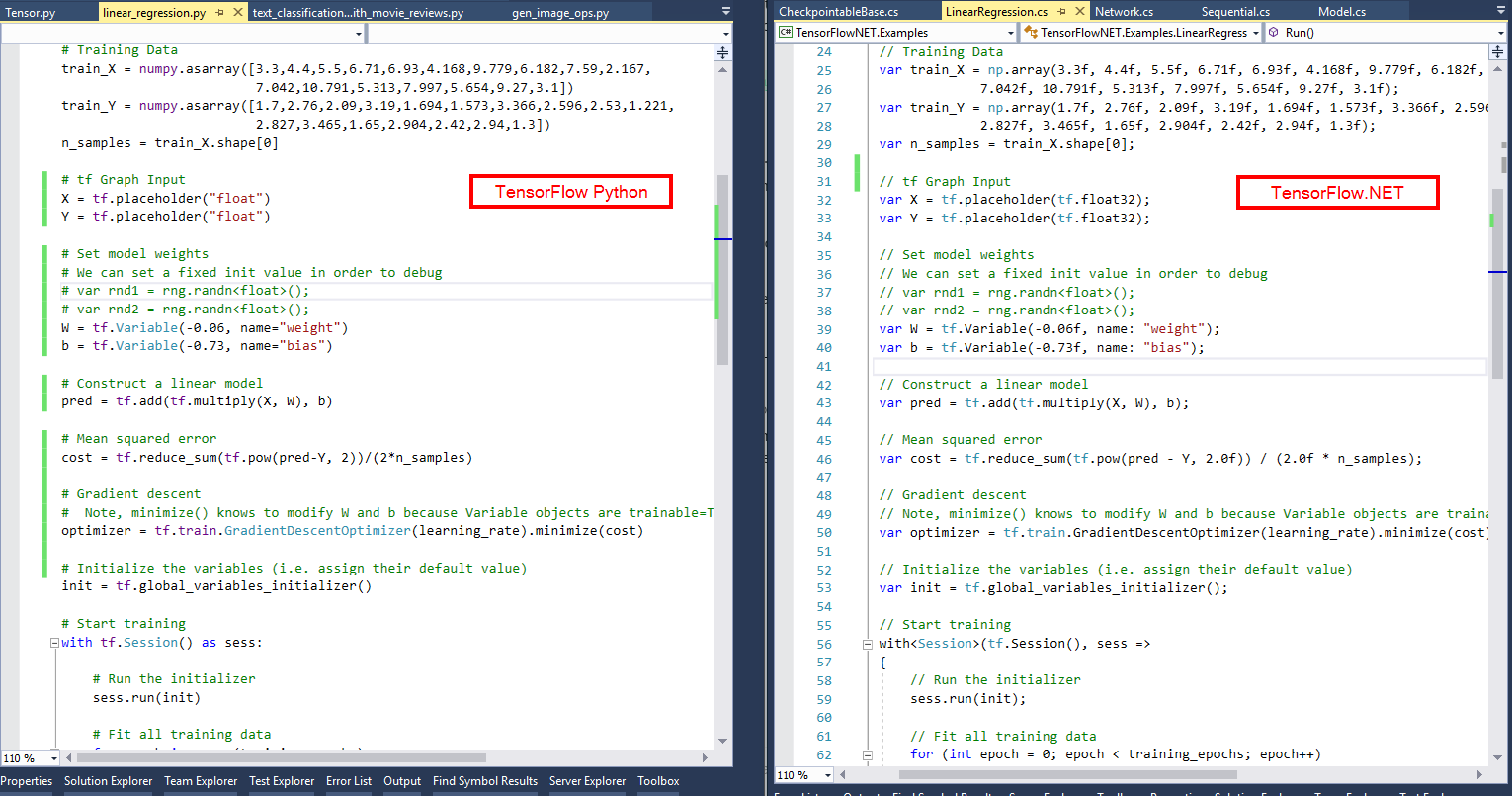# hcchappy / TensorFlow.NET

.NET Standard bindings for Google's TensorFlow for developing, training and deploying Machine Learning models in C#.

https://scisharp.github.io/TensorFlow.NET

Geek Repo

Github PK ToolTensorFlow.NET (TF.NET) provides a .NET Standard binding for TensorFlow. It aims to implement the complete Tensorflow API in C# which allows .NET developers to develop, train and deploy Machine Learning models with the cross-platform .NET Standard framework.

master branch is based on tensorflow 2.3 now, v0.15-tensorflow1.15 is from tensorflow1.15.### Why TensorFlow.NET ?

`SciSharp STACK`'s mission is to bring popular data science technology into the .NET world and to provide .NET developers with a powerful Machine Learning tool set without reinventing the wheel. Since the APIs are kept as similar as possible you can immediately adapt any existing Tensorflow code in C# with a zero learning curve. Take a look at a comparison picture and see how comfortably a Tensorflow/Python script translates into a C# program with TensorFlow.NET.SciSharp's philosophy allows a large number of machine learning code written in Python to be quickly migrated to .NET, enabling .NET developers to use cutting edge machine learning models and access a vast number of Tensorflow resources which would not be possible without this project.

In comparison to other projects, like for instance TensorFlowSharp which only provide Tensorflow's low-level C++ API and can only run models that were built using Python, Tensorflow.NET also implements Tensorflow's high level API where all the magic happens. This computation graph building layer is still under active development. Once it is completely implemented you can build new Machine Learning models in C#.

### How to use

TensorFlow tf 1.13 tf 1.14 tf 1.15 tf 2.2
tf.net 0.20 x x
tf.net 0.15 x x
tf.net 0.14 x x

Install TF.NET and TensorFlow binary through NuGet.

```### install tensorflow C# binding
PM> Install-Package TensorFlow.NET

### Install tensorflow binary
### For CPU version
PM> Install-Package SciSharp.TensorFlow.Redist

### For GPU version (CUDA and cuDNN are required)
PM> Install-Package SciSharp.TensorFlow.Redist-Windows-GPU```

Import TF.NET in your project.

`using static Tensorflow.Binding;`

Linear Regression:

```// Parameters
int training_steps = 1000;
float learning_rate = 0.01f;
int display_step = 100;

// We can set a fixed init value in order to demo
var W = tf.Variable(-0.06f, name: "weight");
var b = tf.Variable(-0.73f, name: "bias");
var optimizer = tf.optimizers.SGD(learning_rate);

// Run training for the given number of steps.
foreach (var step in range(1, training_steps + 1))
{
// Run the optimization to update W and b values.
// Wrap computation inside a GradientTape for automatic differentiation.
using var g = tf.GradientTape();
// Linear regression (Wx + b).
var pred = W * X + b;
// Mean square error.
var loss = tf.reduce_sum(tf.pow(pred - Y, 2)) / (2 * n_samples);
// should stop recording

// Update W and b following gradients.

if (step % display_step == 0)
{
pred = W * X + b;
loss = tf.reduce_sum(tf.pow(pred - Y, 2)) / (2 * n_samples);
print(\$"step: {step}, loss: {loss.numpy()}, W: {W.numpy()}, b: {b.numpy()}");
}
}```

Run this example in Jupyter Notebook.

Read the docs & book The Definitive Guide to Tensorflow.NET.

There are many examples reside at TensorFlow.NET Examples.

Troubleshooting of running example or installation, please refer here.

### Contribute:

Feel like contributing to one of the hottest projects in the Machine Learning field? Want to know how Tensorflow magically creates the computational graph? We appreciate every contribution however small. There are tasks for novices to experts alike, if everyone tackles only a small task the sum of contributions will be huge.

You can:

• Port Tensorflow unit tests from Python to C#
• Port missing Tensorflow code from Python to C#
• Port Tensorflow examples to C# and raise issues if you come accross missing parts of the API
• Debug one of the unit tests that is marked as Ignored to get it to work
• Debug one of the not yet working examples and get it to work

### How to debug unit tests:

The best way to find out why a unit test is failing is to single step it in C# and its pendant Python at the same time to see where the flow of execution digresses or where variables exhibit different values. Good Python IDEs like PyCharm let you single step into the tensorflow library code.

### Git Knowhow for Contributors

Add SciSharp/TensorFlow.NET as upstream to your local repo ...

``````git remote add upstream git@github.com:SciSharp/TensorFlow.NET.git
``````

Please make sure you keep your fork up to date by regularly pulling from upstream.

``````git pull upstream master
``````

### Contact

Feel free to star or raise issue on Github.

Follow us on Medium.

Scan QR code to join Tencent TIM group: